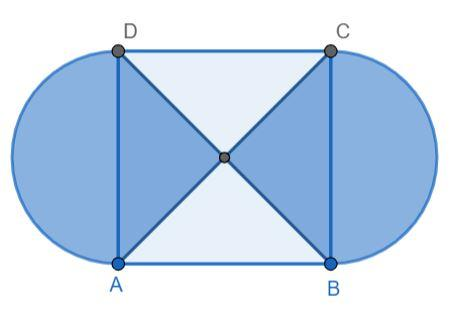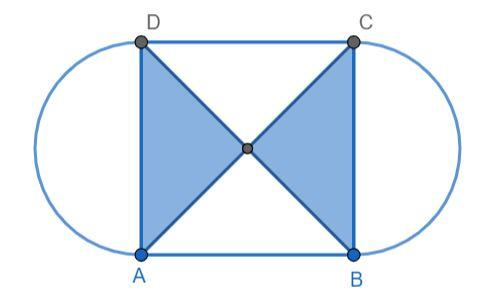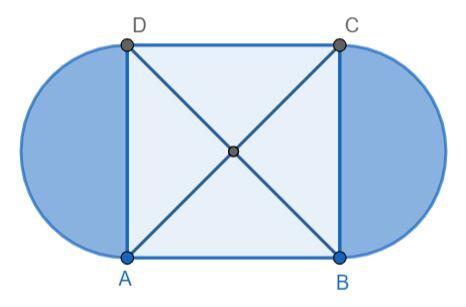Courses
Courses for Kids
Free study material
Offline Centres
MoreLast updated date: 29th Nov 2023
Total views: 379.5k
Views today: 6.79k

# In the given figure, ABCD is a square of side 21 cm. AC and BD are two diagonals of the square. Two semi-circles are drawn with AD and BC as diameters. Find the area of the shaded region (in $c{m^2}$ ). (Take $\pi = \dfrac{{22}}{7}$)Verified
379.5k+ views
Hint: Calculate the area of the shaded region inside the square ABCD and then calculate the area of the two semi-circles and add them both to find the answer.

Let the area of the shaded region inside the square be ${A_1}$ and the area of the shaded region outside the square be ${A_2}$.The two diagonals divide the square into four equal parts. In the figure, two out of the four parts are shaded. Hence, the area of the shaded region inside the square is half of the area of the square. We know that the area of the square of side a is ${a^2}$.

${A_1} = \dfrac{1}{2}{a^2}..........(1)$

It is given that the length of the side of the square is 21 cm. Hence, substituting in equation (1), we get:

${A_1} = \dfrac{1}{2}{(21)^2}$

${A_1} = 220.5c{m^2}...........(2)$

The shaded region outside the square are two semicircles with diameter 21 cm. Hence, the total area is equal to the area of the circle with diameter 21 cm.The area of circle with diameter d is given as follows:

${A_2} = \pi {\left( {\dfrac{d}{2}} \right)^2}............(3)$

Substituting the value d = 21 cm in equation (3), we get:

${A_2} = \pi {\left( {\dfrac{{21}}{2}} \right)^2}$

${A_2} = \dfrac{{22}}{7}{\left( {\dfrac{{21}}{2}} \right)^2}$

${A_2} = \dfrac{{11 \times 21 \times 3}}{2}$

${A_2} = 346.5c{m^2}..........(4)$

The total area is the sum of the two areas we calculated.

$A = {A_1} + {A_2}$

From equations (2) and (4), we have:

$A = 220.5 + 346.5$

$A = 567c{m^2}$

Hence, the area of the shaded region is 567 $c{m^2}$.

Note: You can also find the area of each region separately and calculate the total area. Be careful while calculating the area of the semicircles, 21 cm is the diameter and not the radius.